# A Crop Disease Image Recognition Algorithm Based on Feature Extraction and Image Segmentation

A Crop Disease Image Recognition Algorithm Based on Feature Extraction and Image Segmentation

Chuanzhong MaoWeili Meng Chunying Shi Cuicui Wu Jin Zhang

Laboratory Management Center, Shandong Agriculture and Engineering University, Jinan 250100, China

Corresponding Author Email:
z2013374@sdaeu.edu.cn
Page:
341-346
|
DOI:
https://doi.org/10.18280/ts.370221
17 November 2019
|
Accepted:
9 January 2020
|
Published:
30 April 2020
| Citation

OPEN ACCESS

Abstract:

Due to the complex background of the field, it is a highly complex and flexible task to recognize and diagnose diseases from crop images. Image processing and machine vision can adapt to the complex and changing natural scenes, laying the basis for recognition and diagnosis of crop diseases. This paper designs and verifies an image segmentation method and a disease recognition method for crop disease images under complex background. The segmentation method was developed by improving graph-cut segmentation algorithm with saliency map and excess-green method, while the recognition method was designed based on a single hidden-layer forward neural network (NN). Experimental results show that our segmentation method outperformed he traditional graph-cut algorithm, and fuzzy c-means (FCM) clustering in segmenting the fine-grained disease images, and that our recognition method could accurately identify typical leaf diseases with high stability. The research results provide a good reference for the application of image processing and machine vision in disease image processing.

Keywords:

image recognition, image segmentation, feature extraction, crop diseases

1. Introduction

Crop growth is easily affected by multiple factors, including environment, climate, and soil. These factors complicate the background of crop images. Traditionally, crop diseases are diagnosed and recognized manually. But the manual approach cannot adapt to the complex and changing natural scenes. The problem can be solved effectively by image processing and machine vision, which meet the needs of various applications. It is feasible to diagnose and identify crop diseases through image processing and machine vision. The diagnosis and identification help to establish a disease prediction mechanism, and determine the favorable time for disease prevention and control.

Due to the complex background of the field, it is a highly complex and flexible task to recognize and diagnose diseases from crop images. First, the disease images collected under complex background have high fuzziness: some disease leaves are blocked, and some disease leaves are mixed with disease spots. A series of preprocessing operations is needed to highlight the key information and suppress the useless information in the images, making them suitable for further processing.

Second, the background of crop disease images is very complicated: the leaves often overlap each other and suffer from distortion and deformation; the illumination varies from place to place; the distinction is poor between disease plants, healthy plants, and the soil. This calls for efficient segmentation methods that can effectively sperate the object from the complex background.

Third, the crop diseases cannot be ascertained without sufficient knowledge in crop pathology. The diseases should be diagnosed and identified based on the regularity and stability of disease symptoms. To accurately identify crop diseases, it is necessary to select suitable methods for image segmentation, feature extraction, and feature optimization.

This paper attempts to realize efficient and accurate disease identification and diagnosis, relying on image processing and machine vision. Focusing on crop disease images with complex background, an image segmentation method was designed based on graph-cut algorithm, saliency map, and excess-green method, while a disease recognition method was developed from a single hidden-layer forward neural network (NN). The two methods were verified through contrastive experiments on actual disease images.

2. Literature Review

Under complex background, it is difficult to locate the target area and its boundaries by common image segmentation methods, not to mention achieving desired segmentation effect [1-4]. Based on Mercer theorem, Wang et al.  designed a k-means clustering (KMC) segmentation algorithm, and applied the algorithm to segment the disease spots on corn leaves. Jiang et al.  successfully segmented cucumber disease images with the level set model under complex background. Cheng et al.  segmented rice leaf images robustly and accurately with edge detection algorithm. Through KMC recognition and watershed segmentation, Li et al.  automatically located and counted stripe rust pathogens of wheat. Al-Tarawneh  segmented the disease spots of rapeseed leaves through significance detection, and created a vegetable disease recognition system for greenhouse surveillance videos.

Image segmentation based on saliency map [10, 11] has emerged to reduce the interference of background complexity. For example, Zhuo et al.  proposed a significance detection method based on color difference, in which the significance of each pixel equals the mean color difference between the pixel and the whole image. Based on significance detection, Shao et al.  put forward a four-step image segmentation algorithm: the spatial distance between regions was defined as a weight; the weighted color difference between regions was calculated by the contrast of color histogram; the significance of each region was determined as the total weighted color difference between that regions and other regions; the image was segmented based on the significant regions. Zhu et al.  obtained the regional features through dictionary learning, constructed spatial consistency according to conditional random field, and thus derived the saliency map of the image. These saliency map-based methods extract features of the disease spots, providing a powerful guarantee to segmentation effect.

In the light of color features, Lv et al.  recognized green weeds in the field. With the aid of support vector machine (SVM), Rumpf et al.  identified grape leaf lesions based on such features as color, texture, and shape. According to the shape, texture, and color of disease spots, Sun et al.  trained the disease images of cucumber leaves, and achieved a high recognition accuracy based on the trained images. Xu et al.  classified the images on powdery mildew and rust of wheat, based on features like hue, saturation, value (HSV), and shape. Zhang et al.  recognized diseases on rice leaf images with the SVM, and proved that the SVM is superior to the nearest neighbor (NN) and backpropagation neural network (BPNN) in disease recognition rate. Relying on Bayesian and Fisher discriminant functions, Zhou et al.  constructed a recognition model of tomato leaf diseases, extracted the color, texture, and shape from tomato disease images with the model, and optimized these features through -step discriminant analysis and principal component analysis (PCA), achieving excellent recognition results.

3. Crop Disease Image Segmentation Based on Saliency Map

3.1 Graph-cut segmentation based on saliency map

There is an inherent rule in the disease images collected under complex background: the statistical value of an area in the image applies to the other areas, that is, the features extracted from an area in the image are suitable for the other areas. Therefore, the global statistical properties of a disease image can be obtained locally.

This paper attempts to segment disease images under complex background based on saliency map. The saliency map-based segmentation could eliminate the impact from the complex background, and overcome the defects of traditional segmentation algorithms (e.g. sensitivity to local noises), laying the basis for disease recognition.

As shown in Figure 1, our saliency map-based segmentation algorithm covers three processes: acquiring the saliency map of the original disease image; segmenting the complex background with the saliency map as the mask; segmenting the disease spots.

The saliency map was obtained from the original disease image by saliency map detection strategy. Taking the saliency map as the mask of graph-cut algorithm , the foreground and background of the disease image were separately accurately.

## 1.png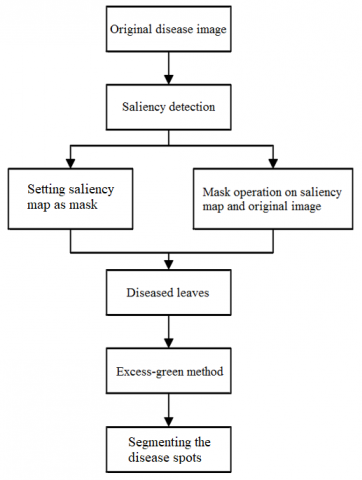Figure 1. The workflow of our saliency map-based segmentation algorithm

In graph-cut algorithm, the foreground and background models are developed based on a k-dimensional Gaussian mixture model (GMM):

$D(x)=\sum_{i=1}^{K} \pi_{i} t_{i}\left(x ; \tau_{i}, \omega_{i}\right)$                 (1)

where, $\sum_{i=1}^{K} \pi_{i}=1 ; \pi$ and $\tau$ are the weight and mean vector of each Gaussian component, respectively.

Our saliency map-based graph cut segmentation method can be implemented in the following steps:

Step 1: Take saliency map as the initial mask $G ;$ initialize background $G_{b},$ empty foreground $G_{f},$ and complement of background $G_{u}=\overline{G_{b}}$ (if $n \in G_{b},$ then $\beta_{n}=0 ;$ if $n \in G_{u},$ then $\left.\beta_{n}=1\right) ;$ establish the GMM of foreground and background with $\beta_{n}=0$ and $\beta_{n}=1$

Step 2:

(1) Calculate GMM parameter $h_{n}$ corresponding to each pixel in $G_{u}$

$h_{n}=\arg \min _{h_{n}} D_{n}\left(a_{n}, h_{n}, \theta, z_{n}\right)$        (2)

(2) Learn the GMM parameter:

$\theta=\arg \min _{\theta} U(a, h, \theta, z)$        (3)

(3) Estimate the segmentation result:

$\min _{\left\{n \in G_{u}\right\}} \min _{h_{n}} E(a, h, \theta, z)$          (4)

Repeat (1) to (3) until the iterative process converges. In each iteration, the GMM parameter and segmentation result are optimized.

Step 3: Update G, and repeat (3) of Step 2.

Step 4: Repeat Step 2.

After the extraction of all diseased leaves, the diseased leaf image was segmented. The leaves disease spots are normally green, while the diseased leaves are usually non-green (e.g. white, gray, brown, and gray black). Therefore, the disease spots were segmented by the excess-green method to extract green color and suppress non-green colors: each pixel of the disease image was compared with a threshold, and categorized to healthy leaves or disease spots. The excess-green method can be defined as:

$E=\left\{\begin{array}{cc}0 & R>G>B \\ 2 G-R-B & \text { other }\end{array}\right.$           (5)

Then, the grayscale image was transformed into a binary image, which was then expanded based on morphology, making the disease spots plumper and natural. Finally, the mask operation was performed on the original disease image to identify the disease spots, and separate them from the leaves.

3.2 Experimental verification

To verify its effectiveness, the graph-cut segmentation method based on saliency map and excess-green method was adopted to process a disease image collected in complex background, where the leaves are similar in color as disease spots under nonuniform illumination. The original image and the segmentation result are given in Figure 2 below.

Next, the proposed segmentation method was further compared with the traditional graph-cut algorithm on a set of standard images and a set of disease images. Table 1 compares the number of iterations and segmentation time for the two methods to achieve the same segmentation results.

## 2.png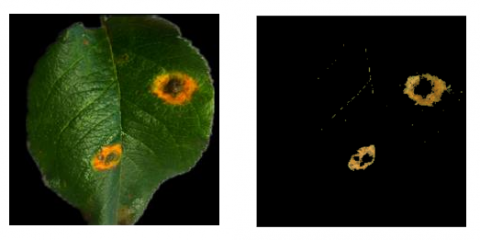Figure 2. Result of disease spots segmentation

As shown in Table 1, our method took 20% less time and 9% fewer iterations than the traditional graph-cut algorithm to achieve the same segmentation results on the standard image set, and 12.5% less time and 27% fewer iterations than the latter to achieve the same segmentation results on the disease image set.

Furthermore, our method, the traditional graph-cut algorithm, and the fuzzy c-means (FCM) clustering were separately applied to process small sets of disease-free leaf images, and leaf images with four types of diseases (gray spot, powdery mildew, gall mite, and anthrax). The mean error rates of the three methods are compared in Table 2.

As shown in Table 2, the FCM clustering had a high mean error rate, indicating that this method failed to remove many background pixels. The traditional graph-cut algorithm achieved a smaller mean error rate than the FCM clustering, but the mean error rate was still above 10%. Our method reduced the mean error rate to below 6%. This means our method could eliminate the background pixels, and fully separate leaves from disease spots. Hence, our method offers the best segmentation strategy among the three contrastive methods.

Table 1. The comparison of the number of iterations and segmentation time

 Traditional graph-cut segmentation algorithm Our method Number of iterations Segmentation time (s) Number of iterations Segmentation time (s) Standard image set 500 14.36 455 11.55 Disease image set 418 12.43 366 9.17

Table 2. The comparison between the three methods in mean error rate

 Disease type FCM clustering Traditional graph-cut algorithm Our method Disease-free 19.6 13.6 6.8 Gray spot 42.7 37.9 7.3 Powdery mildew types 33.9 31.4 7.7 Gall mite 34.7 30.8 3.8 Anthrax 28.9 26.8 2.1
4. Disease Image Recognition Based on Single Hidden-Layer Forward NN

Compared with traditional recognition algorithms, the neural network (NN) can recognize disease spots accurately despite complex background and massive nonlinear data, and clarify the complex relationship between disease image and disease spot. The NN is usually trained by gradient-based learning algorithm, and its weight is often adjusted through error backpropagation. If the learning rate is unreasonable, however, the algorithm will converge very slowly and fall into the local minimum trap. To solve these problems, this paper designs a forward NN with a single hidden layer, and applies it to recognize leaf diseases.

## 3.png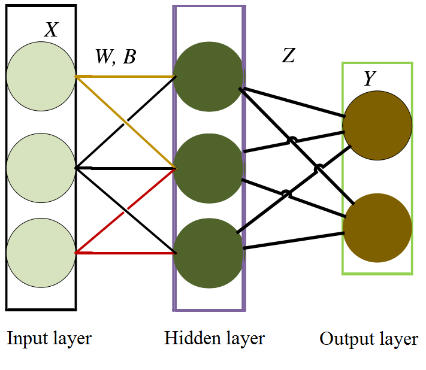Figure 3. The structure of single hidden-layer forward NN

4.1 Single hidden-layer forward NN

As shown in Figure 3, the standard single hidden-layer forward NN consists of an input layer, a hidden layer, and an output layer.

As shown in Figure 3, the training sample X enters the NN via the input layer. In the hidden layer, the sample X is multiplied with weight matrix W, and added with bias B. The sum is imported into the activation function. In the output layer, the result of the activation function is multiplied with weight matrix Z, yielding the output of the single hidden-layer forward NN. The operations in the single hidden-layer NN can be expressed as:

$F(X)=\min _{\boldsymbol{W}, \boldsymbol{B}, \boldsymbol{Z}}\|Z \times H(W X+B)-Y\|$             (6)

where, X is the training sample; H(*) is the activation function; W is the weight matrix of the edges between input and hidden layers; B is the bias between input and hidden layers; Z is the weight matrix of the edges between hidden and output layers; Y is the label of the sample.

In the single hidden-layer forward NN, the weight matrix Z has a unique output, as long as weight W and bias B are determined. The training of the NN is equivalent to solving a linear system. The bias B can be computed by:

$B=H(X W+B)^{\prime} \times Y$   (7)

where, (XW+B)’ is a Penrose generalized inverse matrix.

The common activation functions for the single hidden-layer forward NN include Sigmoid, Hardlim, Tribas, and Radbas.

(1) The limited output range of Sigmoid prevents data from divergence during transmission and facilitates the derivation operation. The Sigmoid activation function can be defined as:

Sigmoid $(\mathrm{x})=1 /(1+\exp (-x))$      (8)

where, exp is exponential operation.

(2) Hardlim outputs 1 if the input reaches the given threshold, and outputs 0 if otherwise. The Hardlim activation function can be defined as:

Hardlim(x) $=\left\{\begin{array}{ll}1, & x>T \\ 0 . & \text { other }\end{array}\right.$        (9)

where, T is the threshold.

(3) Tribas is similar to Gaussian radial basis function (RBF), but has a simple calculation process. The Tribas activation function can be defined as:

Tribas(x) $=\left\{\begin{array}{lr}1-\operatorname{abs}(x), & -1 \leq x \leq 1 \\ 0, & \text { other }\end{array}\right.$            (10)

where, abs(x) is absolute value operation.

(4) Radbas triggers node response with Euclidean distance between samples. The Radbas activation function can be defined as:

Radbas(x) $=\exp \left(-\gamma\|x-\bar{x}\|^{2}\right)$         (11)

where, $\gamma$ is the degree of freedom (DOF); $\bar{x}$ is the mean value.

Table 3. The comparison between single hidden-layer forward NNs with different activation functions

 Activation function Training time Test accuracy Test time Sigmoid 25.3 90.5% 1.07 Hardlim 66.8 90.2% 1.15 Tribas 33.1 45.8% 1.13 Radbas 109.7 35.2% 1.22

Table 3 compares the classification accuracy of single hidden-layer forward NNs with different activation functions, with 100 nodes on the hidden layer.

As shown in Table 3, Sigmoid achieved basically the same accuracy as Hardlim, using only 62% shorter time than the latter. It can be seen that Sigmoid is the best choice for activation function of the single hidden-layer forward NN.

The excellence of Sigmoid stems from the mapping of signals into the range of 0-1 smoothly, so that the signal gain is large for the middle value and small for polar values. Tribas and Radbas also map signals to 0-1, but their derivative functions have inconsistent value symbols. During the processing of our image data, the gradient direction of Tribas and Radbas change abruptly, making it difficult to map the image features. In this case, the NN is pone to non-convergence in training, failing to achieve a good recognition effect.

In practical application, it is neither stable nor robust to classify image datasets with the single hidden-layer forward NN. To recognize leaf diseases, various classifiers were constructed and trained by the sample data. The trained classifiers were fused into a strong classifier. This ensemble algorithm is explained in Figure 4 below.

## 4.png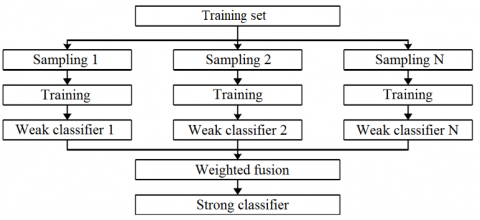Figure 4. The workflow of ensemble algorithm

In the initial phase, the same weight was assigned to each sample, and the single hidden-layer forward NN was adopted to train the samples, producing the base classifier c1(x). According to the preliminary training results of each c1(x) training sample, the weights of the samples trained by the classifier was assigned again, aiming to reduce the weights of correctly classified samples and increase the weights of incorrectly classified samples. In this way, classifier c2(x) and weight w2 were obtained. Similarly, multiple base classifiers and weights were generated iteratively. Then, all base classifiers were integrated by weights into the final strong classifier.

The ensemble algorithm can be implemented in the following steps:

Let $T=\left\{\left(x_{1}, y_{2}\right), \ldots,\left(x_{i}, y_{i}\right)\right\}$ be the training set, where $x_{i}$ is sample and $y_{i}$ is class.

Step 1: Divide the samples into M sets, and initialize the weight of each sample: Wt=1/M.

Step 2: Obtain the base classifier $c_{t}(x): X \rightarrow\{1,2, \ldots, \mathrm{K}\}$ through the learning of the preliminarily weighted dataset.

Step 3: Calculate the classification error rate of $c_{t}(x)$

$e r r_{t}=\sum_{i=1}^{M} W_{t i} \|\left(c_{t}\left(x_{i}\right) \neq y_{i}\right)$     (12)

Step 4: Calculate the weight coefficient of ct(x):

$\beta_{t}=\frac{1}{2} \log \frac{1-e r r_{t}}{e r r_{t}}+\log (K-1)$        (13)

Step 5: Update the centralization value distribution of training data:

$W_{t+1, i}=\frac{W_{t i}}{Z_{t}} \exp \left(-\beta_{t} y_{i} c_{t}\left(x_{i}\right)\right)$          (14)

where, Zt is the standardization factor.

Step 6: Linearly combine the base classifiers into the final strong classifier.

$f(x)=\sum_{t=1}^{M} \beta_{t} c_{t}(x)$         (15)

4.2 Experimental verification

In the experiment, the single hidden-layer forward NN is trained by continuously adjusting the weight and threshold to obtain the minimum performance function; the accuracy is measured by the variance and mean squared error (MSE) between the network output and the actual output. Several effective eigenvalues of the data were extracted and inputted into the NN, and the output of the network was divided into five classes: leaf rust, leaf defoliation, leaf round spot, mosaic, and powdery mildew.

For the single hidden-layer forward NN, there is no clear rule about the number of nodes on the hidden layer. In general, if there are too few hidden layer nodes, the connection weights between input and output layers will be so small that the NN will have a poor learning ability and many errors in the face of complex problems; if there are too many hidden layer nodes, the NN will suffer from over-fitting and poor generalization. Currently, the number of hidden layer nodes is usually set empirically. Table 4 compares the performance of single hidden-layer forward NNs with different number of hidden layer nodes.

As shown in Table 4, with the growing number of hidden layer nodes, the training time and test time of all NNs were on the rise. Meanwhile, the accuracy gain gradually declined. To balance accuracy and efficiency, it is advised that the single hidden-layer forward NN should have 550 hidden layer nodes.

The single hidden-layer forward NNs with different number of hidden layer nodes were also adopted to recognize leaf images on five types of diseases, including leaf rust, leaf defoliation, leaf round spot, mosaic, and powdery mildew. The experimental results are shown in Table 5.

As shown in Table 5, accuracy of disease recognition increased with the number of hidden layer nodes. This is because the number of hidden layer nodes is positively correlated with the number of parameters obtained by the single hidden-layer forward NN. The more the parameters, the better trained the NN, and the higher the accuracy. Among the five diseases, leaf defoliation and mosaic were poorly recognized, for leaf defoliation is similar to leaf round spot in the early phase. By contrast, the powdery mildew was recognized with the highest accuracy, thanks to its obvious color.

Our method was further compared with the SVM in the recognition of leaf round spot, leaf rust, and mosaic. The recognition accuracy of the two methods is compared in Figure 5.

As shown in Figure 5, the leaf disease recognition algorithm based on single hidden-layer forward NN was more accurate than the SVM on diseases like leaf round spot, leaf rust, and mosaic, indicating that our algorithm is a desirable tool in the recognition of leaf diseases.

## 5.png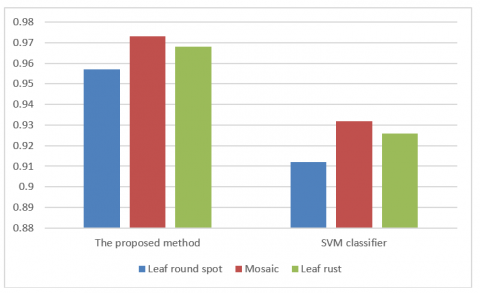Figure 5. The comparison of recognition accuracy

Table 4. The comparison of training and test performance

 Number of hidden layer nodes Training time Test accuracy Test time 150 5.2 93.6% 0.26 350 11.8 94.5% 0.53 550 15.3 95.2% 0.75 750 23.9 96.2% 1.12

Table 5. The comparison of disease recognition accuracy

 Number of hidden layer nodes Leaf rust Leaf defoliation Leaf round spot Mosaic Powdery mildew 150 94.3% 92.2% 93.7% 92.9% 94.5% 350 95.1% 93.1% 94.5% 93.8% 95.2% 550 96.4% 94.1% 96.2% 95.1% 97.8% 750 96.9% 94.7% 96.6% 95.7% 98.2%
5. Conclusions

Focusing on crop disease images under complex background, this paper designs an image segmentation method by improving graph-cut segmentation algorithm with saliency map and excess-green method, and proposes a disease recognition method based on a single hidden-layer forward NN. Experimental results show that our image segmentation method is robust against interferences (e.g. image noises, uneven illumination, and uneven color of disease spots); our algorithm takes fewer iterations and shorter time to achieve the same segmentation effect as the traditional graph-cut algorithm and the FCM clustering. Similarly, the single hidden-layer forward NN was found to outperform the SVM in disease image recognition.

References

 Zheng, L., Zhang, J., Wang, Q. (2009). Mean-shift-based color segmentation of images containing green vegetation. Computers & Electronics in Agriculture, 65(1): 93-98. https://doi.org/10.1016/j.compag.2008.08.002

 Wu, Y., Zhao, L., Jiang, H.Y., Guo, X.Q., Huang, F. (2014). Image segmentation method for green crops using improved mean shift. Transactions of the Chinese Society of Agricultural Engineering, 30(24): 161-167.

 Reddy, U.J., Dhanalakshmi, P., Reddy, P.D.K. (2019). Image segmentation technique using SVM classifier for detection of medical disorders. Ingénierie des Systèmes d’Information, 24(2): 173-176. https://doi.org/10.18280/isi.240207

 Ponti, M.P. (2013). Segmentation of low-cost remote sensing images combining vegetation indices and mean shift. IEEE Geoscience & Remote Sensing Letters, 10(1): 67-70. https://doi.org/10.1109/LGRS.2012.2193113

 Wang, Z.B., Wang, K.Y., Yang, F., Pan, S.H., Han, Y.Y. (2018). Image Segmentation of Overlapping Leaves Based on Chan–Vese Model and Sobel Operator. Information Processing in Agriculture, 5(1): 1-10. https://doi.org/10.1016/j.inpa.2017.09.005

 Jiang, H., Zhang, J., Yuan, Y., He, M.T., Zheng, S.G. (2012). Segmentation of cucumber disease leaf image based on MDMP-LSM. Transactions of the Chinese Society of Agricultural Engineering, 28(21): 142-148.

 Cheng, Y.E., Qian, Y.J., Zhang, Y.G. (2011). Rice edge detection based on canny edge detection algorithm. Computer Systems & Applications, 20(5): 206-209. https://doi.org/10.3969/j.issn.1003-3254.2011.05.048

 Li, Y.Y., Shi, H., Jiao, L.C., Liu, R.C. (2012). Quantum evolutionary clustering algorithm based on watershed applied to SAR image segmentation. Neurocomputing, 87: 90-98. https://doi.org/10.1016/j.neucom.2012.02.008

 Al-Tarawneh, M.S. (2013). An empirical investigation of olive leave spot disease using auto-cropping segmentation and fuzzy C-means classification. World Applied Ences Journal, 23(9): 1207-1211. https://doi.org/10.5829/idosi.wasj.2013.23.09.1000

 Gion, D., Takimoto, H., Kishihara, M. (2013). Interactive Segmentation for Color Image based on color saliency. IEEJ Transactions on Electronics Information & Systems, 133(3): 24-32. https://doi.org/10.1002/ecj.11652

 Wang, T.M., Chen, Y.Y. (2019). A nonlinear tensor-based machine learning algorithm for image classification. Revue d'Intelligence Artificielle, 33(6): 475-481. https://doi.org/10.18280/ria.330611

 Zhuo, L., Hu, X.C., Jiang, L.Y., Zhang, Y. (2016). A color image edge detection algorithm based on color difference. Sensing and Imaging, 17(1): 1-16. https://doi.org/10.1007/s11220-016-0143-6

 Shao, H., Shen, J., Zhang, Z., Liu, H.Y. (2018). Research and analysis of video image target tracking algorithm based on significance. International Journal of High Performance Systems Architecture, 8(1-2): 82-93. https://doi.org/10.1504/IJHPSA.2018.094149

 Zhu, Z.F., Chen, Q., Zhao, Y. (2014). Ensemble dictionary learning for saliency detection. Image and Vision Computing, 32(3): 180-188. https://doi.org/10.1016/j.imavis.2013.12.015

 Lv, X.X., Lv, X.R., Zhang, Z.L. (2010). Study on the segmentation algorithms of tomatoes image based on the color characteristics. Journal of Agricultural Mechanization Research, 1: 30-32.

 Rumpf, T., Mahlein, A.K., Steiner, U., Oerke, E.C., Dehne, H.W., Plumer, L. (2010). Early detection and classification of plant diseases with support vector machines based on hyperspectral reflectance. Computers & Electronics in Agriculture, 74(1): 91-99. https://doi.org/10.1016/j.compag.2010.06.009

 Sun, Y.M., Li, Y.R., Wang, M., Wang, C.Z., Ling, N., Mur, L.A.J., Shen, Q.R., Guo, S.W. (2018). Redox imbalance contributed differently to membrane damage of cucumber leaves under water stress and Fusarium infection. Plant Science, 274: 171-180. https://doi.org/10.1016/j.plantsci.2018.05.025

 Xu, P., Wu, G., Guo, Y., Chen, X.Y., Yang, H.T., Zhang, R.B. (2017). Automatic wheat leaf rust detection and grading diagnosis via embedded image processing system. Procedia Computer Science, 107: 836-841. https://doi.org/10.1016/j.procs.2017.03.177

 Zhang, J., Kong, F., Li, Z., Wu, J.T., Chen, W., Wang, S.W., Zhu, M.S. (2014). Recognition of honey pomelo leaf diseases based on optimal binary tree support vector machine. Transactions of the Chinese Society of Agricultural Engineering, 44(6): 1541-1562. https://doi.org/10.3969/j.issn.1002-6819.2014.19.027

 Zhou, Y.C., Xu, T.Y., Zheng, W., Deng, H.B. (2017). Classification and recognition approaches of tomato main organs based on DCNN. Transactions of the Chinese Society of Agricultural Engineering, 33(15): 219-226.

 Qi, Y., Zhang, G.S., Li, Y. (2018). An auto-segmentation algorithm for multi-label image based on graph cut. Sensing & Imaging, 19(1): 13. https://doi.org/10.1007/s11220-018-0193-z